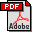Towards an Efficient Tile Matrix Inversion of Symmetric Positive Definite Matrices on Multicore Architectures
Emmanuel Agullo (Dpt of Electrical Engineering and Computer Science, University of Tennessee)
Henricus Bouwmeester (Dpt of Mathematical and Statistical Sciences, University of Colorado Denver)
Jack Dongarra (Dpt of Electrical Engineering and Computer Science, University of Tennessee)
Jakub Kurzak (Dpt of Mathematical and Statistical Sciences, University of Colorado Denver)
Julien Langou (University of Colorado Denver)
Lee Rosenberg (Dpt of Mathematical and Statistical Sciences, University of Colorado Denver)
Abstract:
The algorithms in the current sequential numerical linear algebra libraries (e.g. LAPACK) do not parallelize well on multicore architectures. A new family of algorithms, the tile algorithms, has recently been introduced. Previous research has shown that it is possible to write efficient and scalable tile algorithms for performing a Cholesky factorization, a (pseudo) LU factorization, a QR factorization, and computing the inverse of a symmetric positive definite matrix. In this extended abstract, we revisit the computation of the inverse of a symmetric positive definite matrix. We observe that, using a dynamic task scheduler, it is relatively painless to translate existing LAPACK code to obtain a ready-to-be-executed tile algorithm. However we demonstrate that, for some variants, non trivial compiler techniques (array renaming, loop reversal and pipelining) need then to be applied to further increase the parallelism of the application. We present preliminary experimental results.
Keywords:
Numerical Algorithms for CS&E, Numerical Algorithms for Multicore ArchitecturesDownload the fullpaper